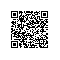# 笔试题目“翻转字符串”的实现

## 笔试题目

//写一个函数，将字符串翻转，翻转方式如下：“I am a student”反转成“student a am I”，不借助任何库函数。

## 算法

#include <stdio.h>

void main()
{
char str[]="I am a student";
printf(str);
printf("\n");

char *p,*q;
char temp;
p=q=str;14     while(*q!='\0')
{
q++;
}
q--;
while(p<=q)
{
temp=*p;
*p=*q;
*q=temp;
p++;
q--;
}//反转整个字符串

printf(str);
printf("\n");

q=str;//指针指向开始位置
char *s,*t;
s=t=str;
while(*q!='\0')
{
if(*q==' ')
{
t--;
while(s<=t)
{
temp=*t;
*t=*s;
*s=temp;
s++;
t--;
}//反转局部字符串

s=q+1;
t=q;
}52         q++;
t++;
}

printf(str);
printf("\n");
}

## 改进if(*q==' ')

if(*q==' '||*(q+1)=='\0')
{
t--;
if(*(q+1)=='\0')//处理最后一个字串
t++;## 代码已处理最后字串的完整代码《程序员面试宝典》实现方法使用钉钉扫一扫加入圈子
+ 订阅